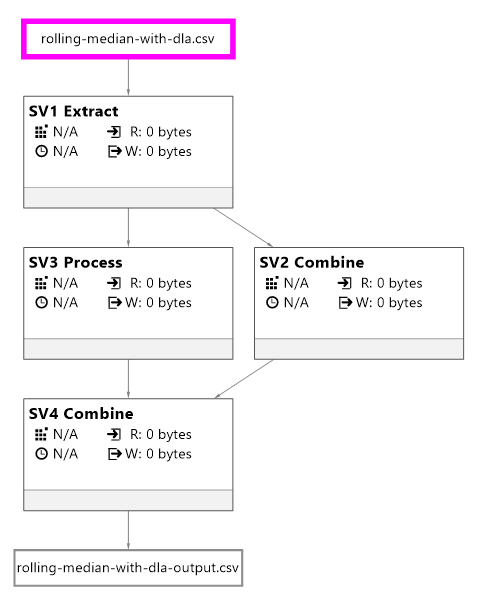Azure Data Lake Analytics (DLA in short) provides a rich set of analytics functions to compute an aggregate value based on a group of rows. The typical example is with the rolling average over a specific window. Below is an example where the window is centered and of size 11 (5 preceding, the current row, and 5 following). The grouping is made over the `site` field.

However, the median function does not support the `ROWS` option. It is not possible therefore to run rolling median straight out of the box with DLA.

The aim of this post is to:

• show you how the running median can be calculated on ADL by using a mix of basic `JOIN`
• discuss the finer points of the median functions in ADL
• compare a R implementation

# Rolling Median with DLA

Let’s generate random time series to illustrate the implementation. The series are here generated with R as follows:

• the data appears every 5 minutes.
• the data is related to different sites as it will help us to illustrate the partition with the DLA analytic queries.
• For each of the site, we generate 100 random values.

The data frame is then saved into a CSV file.

The file is then loaded into DLA with an `EXTRACT` statement. Note that we ensure that the time stamps are all UTC.

This statement outlines how the rolling average will be calculated. Note that the window is centered and should be a odd number (11 in our case). Odd numbers are easier to deal with especially with the median later on.

This is the key piece of code. We are performing a self-join. For each of the current record in the `c` table, we are joining the windowed records (in table `w`) by restricting the window through the `BETWEEN` operator.

Note the use of the `DISTINCT` operator as the `PERCENTILE` functions do not group the rows.

The continuous and discrete operators are used; more on that later.

The `OUTPUT` statement just save the results. Let’s analyse them.

# Reconciliation with R

The script is executed locally (it is a nice feature of DLA as it speed up the development of scripts as opposed as running the script from Azure). The output file can be found here.Let’s make sure that the results are consistent with what we would have found with other means. For example, in R, the `rollapply` function with the `partial` parameter set to `TRUE` replicate the behavior of DLA. If `partial` is set to `FALSE` without padding (see `na.pad`), the first rows of each group will be removed as the data does not fit the window.

A quick reconciliation with DLA (represented by the data frame `d.dla`) indicate that the values are similar.

## Note

There are differences between the rolling average in R and in DLA for the last 5 records of each group. I have not figured out why yet; I will submit the issue on stackoverflow.

# `PERCENTILE_DISC` vs `PERCENTILE_CONT`

It is worth noting that `PERCENTILE_DISC` (the discrete implementation of the median) is different with the `PERCENTILE_CONT` for the first 5 rows of each group.

If we look at the first value with a centered window of size 11, only the current row and the next 5 rows will be taken into account. So, with the continuous median, the value is the middle between position 3 and 4 of the sorted values:

With the discrete mean in ADL, it is the position 3 of the sorted vector.